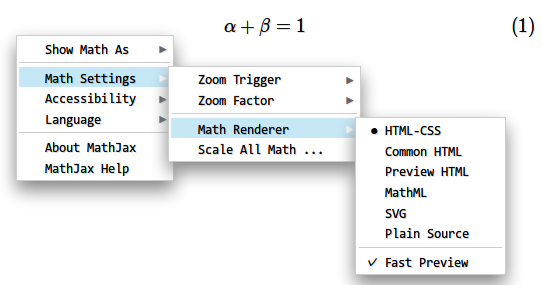# latex公式编号在输出到html时没有显示编号

\begin{equation} \alpha + \beta = 1 \end{equation}

Org-mode version 8.2.10

#+TITLE: my notes #+AUTHOR: mycyber #+LATEX_CLASS: article #+LATEX_CLASS_OPTIONS: [a4paper] #+LATEX_HEADER: \usepackage[utf8]{ctex} #+LATEX_HEADER: \usepackage[bottom=2cm]{geometry}

\begin{equation} \alpha + \beta = 1 \end{equation}